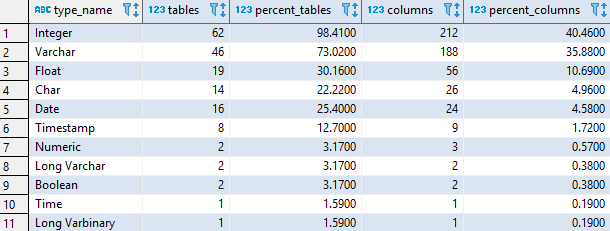## Find most used data type in Vertica database

Article for:

Query below returns data types used in a database ordered by the number of their occurance.

## Query

``````with sum_all as(
select count(distinct table_id) as tables,
count(column_name) as columns
from v_catalog.columns
)
select t.type_name,
count(distinct c.table_id) as tables,
100*round(count(distinct c.table_id)/ sums.tables,4) as percent_tables,
count(*) as columns,
100*round(count(*)/ sums.columns, 4) as percent_columns
from v_catalog.columns c
join v_catalog.types t
on c.data_type_id = t.type_id
join sum_all sums on true
group by t.type_name,
sums.tables,
sums.columns
order by columns desc,
tables desc;
``````

## Columns

• type_name - built in or user data type without length or precision, e.g. int, varchar or date
• tables - number of tables in a database with this data type
• percent_tables - percentage of tables with columns with this data type.
• columns - number of columns in a database with this data type
• percent_columns - percentage of columns with this data type. Rows add up to 100%.

## Rows

• One row represents one data type
• Scope of rows: all data types used in a database
• Ordered by number of columns descending, number of tables descending

## Sample results